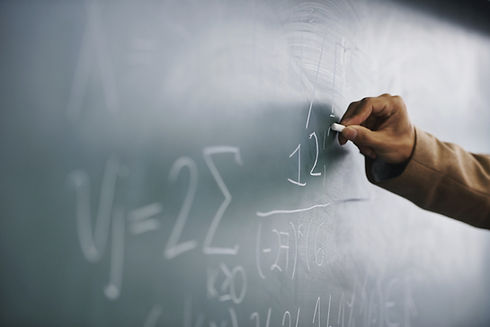top of page# MATH PUZZLES

Xaver Blackwell-Lipkind

Note: The problems below, listed in no particular order, represent a wide range of difficulties. All are original.

1. In the following recursive sequence, the first term is given. Write an equation representing the sequence. You may not use the modulus operation or any type of floor, step, or conditional function.

1, 1, 2, 2, 3, 3, 4, 4,...

2. Find a reliable method of discerning powers of eleven from Pascal’s triangle. It is not correct to claim that the rows of Pascal’s triangle directly represent the powers of eleven. What happens when two-digit numbers begin to appear? What about three-digit numbers? What about n-digit numbers?

3. Find the derivative of the function f(x) = x^x without any sort of calculator. Hint: the derivative can be found easily after rearranging the equation into a slightly different format.

4. What is the simplest method of computing the product 6003.5 * 5996.5 without a calculator?

5. Prove that the sum of the first n primes will always be odd when n is even and even when n is odd.

bottom of page